# Репетитор Лингвистического Университета - МГЛУ - Репетитор по английскому языку и математике на англ. яз. в Москве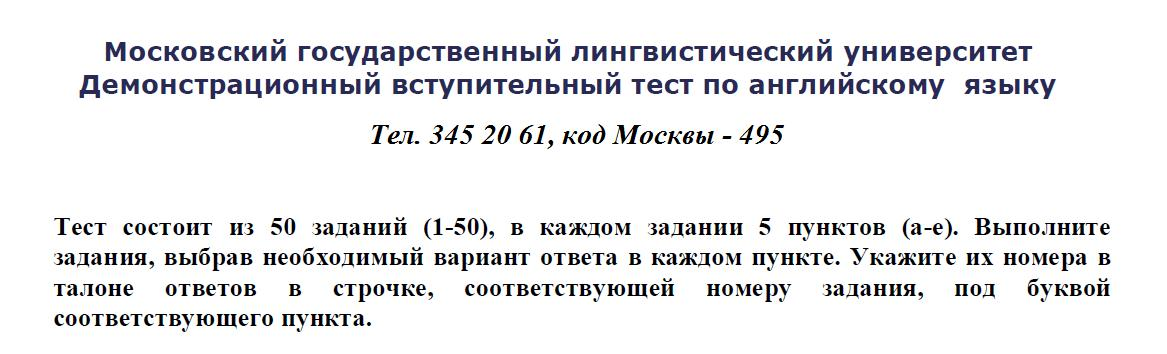## Частные уроки. Тесты вступительных экзаменов в МГЛУ - Московский государственный лингвистический университет - скачать бесплатно - download free of charge

Пример запроса с просьбой прислать правильные ответы на вопросы билетов теста:

Добрый день, Алексей!
Пришлите, пожалуйста, правильные ответы с комментариями и объяснениями да заодно и сами варианты ответов на вступит. контрольную работу - билеты дополнительных вступительных экзаменов МГЛУ (бывший Институт иностранных языков им. Мориса Тореза)
по Скайпу или на мой электронный почтовый адрес. Как Вам удобнее.
Могу ли я оплатить, пополнив счет телефона?
Абитуриентка МГЛУ - Московского Государственного Лингвистического Университета - 2012 года.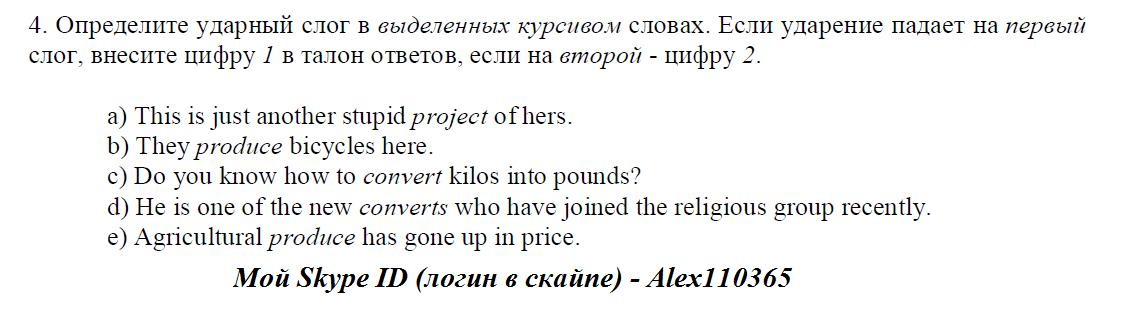Цена - на Arepetitor.ru

Телефон в Москве - 345 20 61, моб. 8-917 531 85 49.

М. Марьино.

Алексей Эдвардович.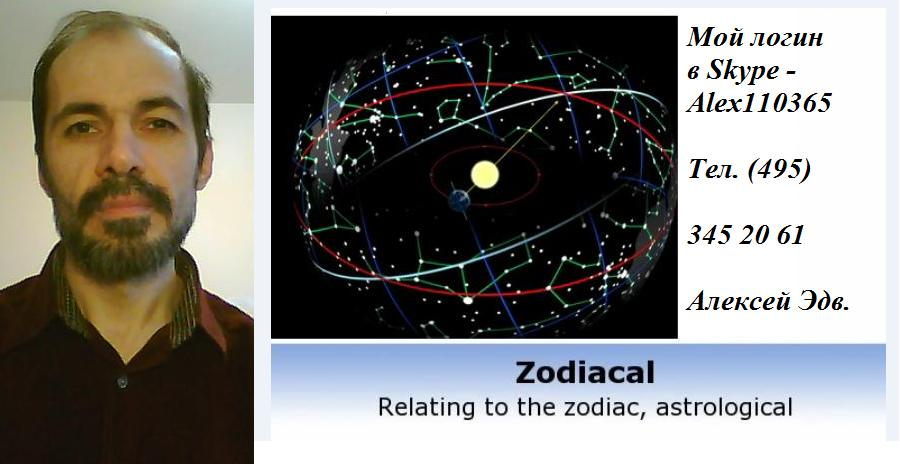### Пример переписки в скайпе по поводу уроков математики на англ. яз.:

- Алексей Эдуардович!

В дополнение к нашему телефонному разговору о репетиторстве.
Файл учебника в формате PDF прилагаю к письму.
Прошу позвонить после того, как Вы откроете и посмотрите учебник по теории вероятности и статистике на английском языке.
Все подробности можно обсудить по телефону, мой мобильный 8-916 828 14 65.

Алексей Султанов

### Exercises and Complements

1.1.1 (Example 1.1.1 Continued) In the set up of the Example 1.1.1, find the probability of observing no heads or two heads.

1.1.2 (Example 1.1.2 Continued) In the set up of the Example 1.1.2, find the probability of observing a difference of one between the scores which come up on the two dice. Also, find the probability of observing the red die scoring higher than that from the yellow die.

1.1.3 Five equally qualified individuals consisting of four men and one woman apply for two identical positions in a company. The two positions are filled by selecting two from this applicant pool at random.

(i) Write down the sample space S for this random experiment;

(ii) Assign probabilities to the simple events in the sample space S;

(iii) Find the probability that the woman applicant is selected for a position.

1.2.1 One has three fair dice which are red, yellow and brown. The three dice are rolled on a table at the same time. Consider the following two events:

A : The sum total of the scores from all three dice is 10

B : The sum total of the scores from the red and brown dice exceeds 8

Find P(A), P(B) and P(A n B).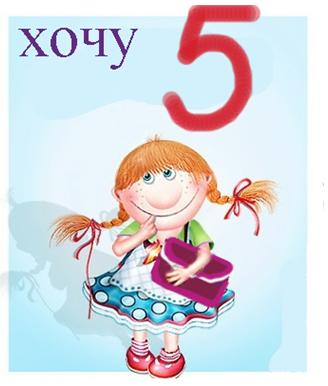1.2.2 Prove the set relations given in (1.2.2).

1.2.3 Suppose that A, B, C are subsets of S. Show that

(i) A Δ C ⊆ (A Δ B) ∪ (B Δ C);

(ii) (A Δ B) ∪ (B Δ C)

= [(A B) ∩ (A B)c] ∪ [(B C) ∩ (B C)c].

{Hint: Use the Venn Diagram.}

1.2.4 Suppose that A1, ..., An are Borel sets, that is they belong to ß. Define the following sets: B1 = A1, B2 = A2 ∩ B3 = A3 ∩ (A1 ∪ A2)c, ..., Bn = An ∩ (A1 ∪ ... ∪ An.1) c. Show that

(i) B1, ..., Bn are Borel sets;

(ii) B1, ..., Bn are disjoint sets;

1.2.5 Suppose that S = {(x, y) : x2 + y2 ≤ 1}.
Extend the ideas from the Example 1.2.3 to obtain a partition of the circular disc, S.
{Hint: How about considering Ai = {(x, y) : 1/2i < x2 + y2 = 1/2i.1}, i = 1, 2, ...? Any other possibilities?}

British English - a tutor with experience.

1.3.1 Show that the Borel sigma-field ß is closed under the operation of
(i) finite intersection, (ii) countably infinite intersection, of its members.
{Hint: Can DeMorgan’s Law (Theorem 1.2.1) be used here?}

1.3.2 Suppose that A and B are two arbitrary Borel sets. Then, show that A Δ B is also a Borel set.

1.3.3 Suppose that A1, ..., Ak are disjoint Borel sets. Then show that. Contrast this result with the requirement in part (iii) of the Definition 1.3.4.

1.3.4 Prove part (v) in the Theorem 1.3.1.1.3.5 Consider a sample space S and suppose that ß is the Borel sigma field of subsets of S. Let A, B, C be events, that is these belong to ß. Now, prove the following statements:

(i) P(A B) = P(A) + P(B);

(ii) P(A B) = P(A) + P(B) . 1;

(iii) P(A C) = min{P(A), P(C)};

(iv) P(A B C) = P(A) + P(B) + P(C) . P(A B). P(A C) . P(B C) + P(A B C).

{Hint: Use the Theorem 1.3.1 to prove Parts (i)-(iii). Part (iv) should follow from the Theorem 1.3.1, part (iv). The result in part (ii) is commonly referred to as the Bonferroni inequality. See the Theorem 3.9.10.}

1.3.6 (Exercise 1.3.5 Continued) Suppose that A1, ..., An are Borel sets, that is they belong to ß. Show that.{Hint: Use mathematical induction and part (iv) in the Exercise 1.3.5.}

1.3.7 (Exercise 1.3.5 Continued) Suppose that A1, ..., An are Borel sets, that is they belong to ß. Show that. This is commonly referred to as the Bonferroni inequality.
{Hint: Use mathematical induction and part (ii) in the Exercise 1.3.5. See the Theorem 3.9.10.}

1.3.8 Suppose that A1, A2 are Borel sets, that is they belong to ß. Show that where recall from (1.2.1) that AA2 stands for the symmetric difference of A1, A2.

1.3.9 Suppose that A1, A2, A3 are Borel sets, that is they belong to ß. Recall from (1.2.1) that AiΔ Aj stands for the symmetric difference of Ai, Aj. Show that

(i) AA3 ⊆ (AA2) ∪ (AA3);

(ii) P(AA3) ≤ P(AA2) + P(AA3).

1.4.1 Prove the Theorem 1.4.1.

Online Tutor - English Teacher - TOEFL, IELTS, GMAT, FCA.
Interested in learning English from a teacher? I have a clear English accent and have experience with students of all ages.

1.4.2 In the Theorem 1.4.2, prove that (ii) ⇒ (iii) ⇒ (iv).

1.4.3 Consider a sample space S and suppose that A, B are two events which are mutually exclusive, that is, A B = ϕ. If P(A) > 0, P(B) > 0, then can these two events A, B be independent? Explain.

1.4.4 Suppose that A and B are two events such that P(A) = .7999 and P(B) = .2002. Are A and B mutually exclusive events? Explain.

1.4.5 A group of five seniors and eight first year graduate students are available to fill the vacancies of local news reporters at the radio station in a college campus. If four students are to be randomly selected from this pool for interviews, find the probability that at least two first year graduate students are among the chosen group.1.4.6 Four cards are drawn at random from a standard playing pack of fifty two cards. Find the probability that the random draw will yield

(i) an ace and three kings;

(ii) the ace, king, queen and jack of clubs;

(iii) the ace, king, queen and jack from the same suit;

(iv) the four queens.

1.4.7 In the context of the Example 1.1.2, let us define the three events:

E : The sum total of the scores from the two up faces exceeds 8

F : The score on the red die is twice that on the yellow die

G : The red die scores one point more than the yellow die

Are the events E, F independent? Are the events E, G independent?

1.4.8 (Example 1.4.7 Continued) In the Example 1.4.7, the prevalence of the disease was 40% in the population whereas the diagnostic blood test had the 10% false negative rate and 20% false positive rate. Instead assume that the prevalence of the disease was 100p% in the population whereas the diagnostic blood test had the 100α% false negative rate and 100β% false positive rate, 0 < p, α, β < 1. Now, from this population an individual is selected at random and his blood is tested. The health professional is informed that the test indicated the presence of the particular disease. Find the expression of the probability, involving p, α and β, that this individual does indeed have the disease. {Hint: Try and use the Beyes.s Theorem.}

1.4.9 Let us generalize the scenario considered in the Examples 1.4.5-1.4.6. The urn #1 contains eight green and twelve blue marbles whereas the urn #2 has ten green and eight blue marbles. Suppose that we also have the urn #3 which has just five green marbles. These marbles have the same size and weight. Now, the experimenter first randomly selects an urn, with equal probability, and from the selected urn draws one marble at random. Given that the selected marble was green, find the probability that

(i) the urn #1 was selected;

An English Tutor Explains British and American English
Did you know that many times when a native English speaker talks to a native British speaker, they have difficulty understanding?
Репетитор английского языка.За 26 лет работы я, как репетитор английского языка из МГЛУ, накопил опыт и разработал систему преподавания английского языка, которая позволяет обеспечить высокие баллы на ЕГЭ по английскому языку.
Преподаватель английского языка МГЛУ - Лингвист - Репетитор по английскому языку.

(ii) the urn #2 was selected;

(iii) the urn #3 was selected.

{Hint: Try and use the Bayes Theorem.}

1.4.10 (Exercise 1.2.1 Continued) One has three fair dice which are red, yellow and brown. The three dice are rolled on a table at the same time. Consider the following events:

A : The total score from all three dice is 10

B : The total score from the red and brown dice exceeds 8

Are A, B independent events?

1.4.11 (Example 1.4.4 Continued) Show that P(A) = P(B) assuming that there are respectively m, n green and blue marbles in the urn to begin with. This shows that the result P(A) = P(B) we had in the Example 1.4.4 was not just a coincidence after all.

1.4.12 Show that . {Hint: Can the binomial theorem, namely (a+b)n = , be used here?}

1.4.13 Suppose that we have twenty beads of which eight are red, seven are green and five are blue. If we set them up in a row on a table, how many different patterns are possible?

1.4.14 Suppose that four boys and four girls are waiting to occupy a chair each, all placed in a row adjacent to each other. If they are seated randomly, what is the probability that the boys and girls will alternate? {Hint: The total possible arrangement is 8!. Note that each arrangement could start with a boy or a girl.}

1.4.15 Suppose that we have n different letters for n individuals as well as n envelopes correctly addressed to those n individuals.
If these letters| are randomly placed in these envelopes so that exactly one letter goes in an envelope, then what is the probability that at least one letter will go in the correct envelope?
Obtain the expression of this probability when we let n go to ∞.

{Hint: Let Ai be the event that the ith letter is stuffed in its correct envelope, i = 1, ..., n.
We are asked to evaluate P(A1 ∪ ... ∪ An). Apply the result from the Exercise 1.3.6.
Observe also that P(Ai) = (n.1)!/n!, P(Ai Aj) = (n.2)!/n!, P(Ai Aj Ak) = (n.3)!/n!, ..., P(A1 ∩ ... ∩ An) = 1/n!, for i j k... .
Now all there is left is to count the number of terms in the single, double, triple sums and so on. The answer for P(A1 ∪...∪ An) should simplify to 1 . 1/2! + 1/3! . ... + (.1)n.1 1/n!
which is approximately 1 . e.1 for very large n. See (1.6.13).}

British English tutor - 25 years experience - School - University
US qualified tutor - Excelllent service, competitive rates and all materials supplied. All levels - primary school to adult can be catered.
Новое для Вас слово?
to cater for the best public — обслуживать избранную публику
this playground caters for children of all ages — эта игровая площадка предназначена для детей всех возрастов

TOEFL iBT, SAT, IELTS, GMAT, FCA. Подготовка в зарубежные университеты.

1.4.16 Consider the digits 0, 1, ..., 9. Use these digits at random to form a four (five) digit number. Then, find the probability of forming
(i) a four digit random number, not starting with a zero, which would be an even number while each digit appears exactly once;

(ii) a four digit random number which would be an even number where the digits can be repeated, and starting with a zero is allowed;

(iii) a five digit random number, not starting with a zero, which would be divisible by the number five while each digit appears only once.

1.4.17 In a twin engine plane, we are told that the two engines (#1, #2) function independently. We are also told that the plane flies just fine when at least one of the two engines are working. During a flying mission, individually the engine #1 and #2 respectively may fail with probability .001 and .01.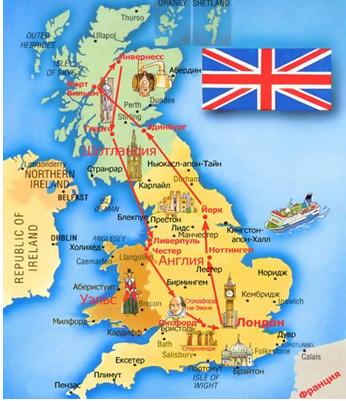Then, during a flying mission, what is the probability that the plane would crash? The plane would complete its mission?

1.4.18 Suppose that A1, ..., Ak are disjoint events. Let B be another event. Then, show that.

1.4.19 Suppose that A1, A2 are events. Then, show that.

1.4.20 Suppose that A1, A2 are disjoint events. Then, show that.

(i) P(A1 | )= P(A1)/{1 . P(A2)} if P(A2) ≠ 1;

(ii) P(A1 | A1 ∪ A2) = P(A1)/{P(A1) + P(A2)}.

1.5.1 Suppose that a random variable X has the following pmf:  X values: .2 0 1 3 8

Probabilities: .2 p .1 2p .4 where p ∈ (0, .1].

(i) Is it possible to determine p uniquely?

(ii) Find P{|X . .5| > 2} and P{|X . .5| = 2.5}.

1.5.2 Suppose that a random variable X has the following pmf: X values: .2 0 1 3 8 Probabilities: .2 p .1 .3 . p .4 where p ∈ (0, .3). Is it possible to determine p uniquely? With a fixed but arbitrary p ∈ (0, .3), find P{|X . .5| > 2}, P{X2 < 4} and P{|X . .3| = 1.7}.

1.5.3 Suppose that a random variable X has the following pmf: X values: .4 .2 1 3 6 Probabilities: .3. p2 p2 .1 2p .4 where p2 ≤ .3. Is it possible to determine p uniquely?

1.5.4 (Example 1.5.2 Continued) Use the form of the df F(x) from the Example 1.5.2 and perform the types of calculations carried out in (1.5.10).

1.5.5 A large envelope has twenty cards of same size. The number two is written on ten cards, the number four is written on six cards, and the number five is written on the remaining four cards. The cards are mixed inside the envelope and we go in to take out one card at random. Let X be the number written on the selected card.

(i) Derive the pmf f(x) of the random variable X;

(ii) Derive the df F(x) of the random variable X;

(iii) Plot the df F(x) and check its points of discontinuities;

(iv) Perform the types of calculations carried out in (1.5.10).

1.5.6 Prove the Theorem 1.5.1.

1.5.7 An urn contains m red and n blue marbles of equal size and weight. The marbles are mixed inside the urn and then the experimenter selects four marbles at random. Suppose that the random variable X denotes the number of red marbles selected. Derive the pmf of X when initially the respective number of marbles inside the urn are given as follows:

(i) m = 5, n = 3;

(ii) m = 3, n = 5;

(iii) m = 4, n = 2.

In each case, watch carefully the set of possible values of the random variable X wherever the pmf is positive. Next write down the pmf of X when m and n are arbitrary. This situation is referred to as sampling without replacement and the corresponding distribution of X is called the Hypergeometric distribution.

Британский английский язык - репетитор со стажем.

1.5.8 A fair die is rolled n times independently while we count the number of times (X) the die lands on a three or six. Derive the pmf f(x) and the df F(x) of the random variable X. Evaluate P(X < 3) and P(|X . 1| > 1).

1.6.1 Let c be a positive constant and consider the pdf of a random variable X given by Figure 1.8.1 gives a plot of f(x) when c = 4/3. It is implicit that x, f(x) are respectively plotted on the horizontal and vertical axes.

Find the correct value of c. Find the df F(x) and plot it. Does F(x) have any points of discontinuity? Find the set of points, if non-empty, where F(x) is not differentiable. Calculate P(. .5 < X ≤ .8). Find the median of this distribution.

Figure 1.8.1. The PDF f(x) from the Exercise 1.6.1 with c = 4/3Сайт управляется системой uCoz